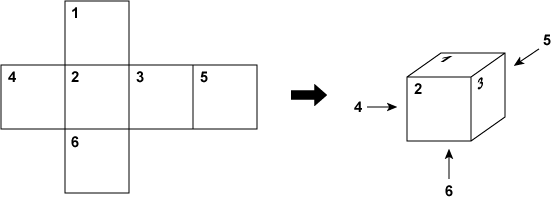# Structure and Class - Dice I

Time Limit : 1 sec, Memory Limit : 131072 KB
Japanese version is here

# Dice I

Write a program to simulate rolling a dice, which can be constructed by the following net.As shown in the figures, each face is identified by a different label from 1 to 6.

Write a program which reads integers assigned to each face identified by the label and a sequence of commands to roll the dice, and prints the integer on the top face. At the initial state, the dice is located as shown in the above figures.

## Input

In the first line, six integers assigned to faces are given in ascending order of their corresponding labels.

In the second line, a string which represents a sequence of commands, is given. The command is one of 'E', 'N', 'S' and 'W' representing four directions shown in the above figures.

## Output

Print the integer which appears on the top face after the simulation.

## Constraints

• $0 \leq$ the integer assigned to a face $\leq 100$
• $0 \leq$ the length of the command $\leq 100$

## Sample Input 1

1 2 4 8 16 32
SE


## Sample Output 1

8


You are given a dice where 1, 2, 4, 8, 16 are 32 are assigned to a face labeled by 1, 2, ..., 6 respectively. After you roll the dice to the direction S and then to the direction E, you can see 8 on the top face.

## Sample Input 2

1 2 4 8 16 32
EESWN


## Sample Output 2

32